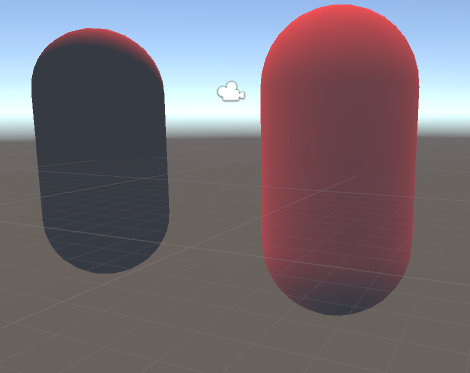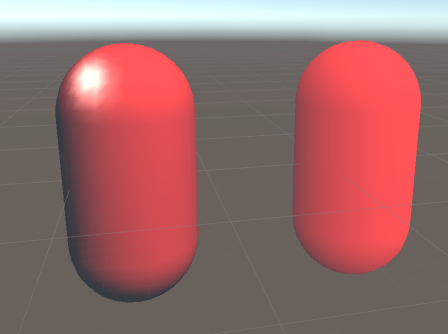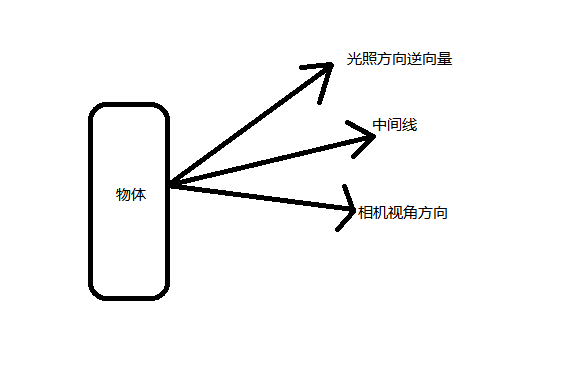Diffuse = 直射光颜色 *（cosθ*0.5+0.5）

Diffuse = 直射光颜色 * max(0,cosθ) θ是指光照方向与法线方向的夹角## 高光反射的计算 (Blinn光照模型)

### Specular = 直射光*pow(max(cosθ,0),高光的参数)

θ是指反射光方向和反射点到相机方向的夹角
pow的次方的意思 就是cosθ的高光参数次方
max(cosθ,0)作用是防止在物体背光面，如果高光参数为偶数，那么最后的值会从负数变为正数

Properties{
_Diffuse("Diffuse Color",Color) = (1,1,1,1)
_Gloss("Gloss",Range(8,200)) = 10
}
Pass{

Tags{"LightMode" = "ForwardBase"}

CGPROGRAM

#include "Lighting.cginc"
//unity系统定义好的类  可以使用一些内置的变量来简化计算操作
//_LightColor0              该变量表示第一个直射光的颜色
//_WorldSpaceLightPos0      该变量表示第一个直射光的位置(世界坐标下)
//UNITY_LIGHTMODEL_AMBIENT  该变量表示系统的环境光
#pragma vertex vert

#pragma fragment frag

fixed4 _Diffuse;
half _Gloss;

struct a2v {
float4 vertex : POSITION; //系统自动将顶点坐标传入vertex
float3 normal : NORMAL;  //系统自动将法线传入    变量normal
};

struct v2f {
float4 position:SV_POSITION;
fixed3 color : COLOR;
};

v2f vert(a2v x) {
v2f f;
//UNITY_MATRIX_MVP 这个矩阵用来把一个坐标从模型空间转换到剪裁空间
f.position = mul(UNITY_MATRIX_MVP, x.vertex);
fixed3 lightDir = normalize(_WorldSpaceLightPos0.xyz);

fixed3 ambient = UNITY_LIGHTMODEL_AMBIENT.rgb;

fixed3 normalDir = normalize(mul(x.normal, (float3x3)unity_WorldToObject));
//暂时没有考虑alpha属性
fixed3 diffuse = _LightColor0.rgb*max(dot(lightDir, normalDir),0)*_Diffuse.rgb;//取得漫反射的颜色

//计算高光
//计算反射光夹角
fixed3 reflectDir = normalize(reflect(-lightDir,normalDir));
fixed3 viewDir = normalize(_WorldSpaceCameraPos.xyz - mul(x.vertex, (float3x3)unity_WorldToObject).xyz);

fixed3 specular = _LightColor0.rgb*pow(max(dot(reflectDir, viewDir), 0), _Gloss);

f.color = diffuse +ambient+ specular;
return f;
}

fixed4 frag(v2f f) :SV_TARGET{
//将计算得到的颜色值输出到视野中的物体上
return fixed4(f.color, 1);
}
ENDCG
}
}_Gloss("Gloss",Range(8,200)) = 10

Blinn-Phone光照模型 :Blinn光照模型的改进

### Specular = 直射光*pow(max(cosθ,0),高光的参数)

θ这里是指法线和x的夹角 x是光的方向和摄像机视角方向的平分线(中间线)v2f vert(a2v x) {
v2f f;
//UNITY_MATRIX_MVP 这个矩阵用来把一个坐标从模型空间转换到剪裁空间
f.position = mul(UNITY_MATRIX_MVP, x.vertex);
fixed3 lightDir = normalize(_WorldSpaceLightPos0.xyz);

fixed3 ambient = UNITY_LIGHTMODEL_AMBIENT.rgb;

fixed3 normalDir = normalize(mul(x.normal, (float3x3)unity_WorldToObject));
//暂时没有考虑alpha属性
fixed3 diffuse = _LightColor0.rgb*max(dot(lightDir, normalDir),0)*_Diffuse.rgb;//取得漫反射的颜色

//计算高光
//计算反射光夹角
//fixed3 reflectDir = normalize(reflect(-lightDir,normalDir));
fixed3 viewDir = normalize(_WorldSpaceCameraPos.xyz - mul(x.vertex, (float3x3)unity_WorldToObject).xyz);

//平分线
fixed3 halfDir = normalize(viewDir + lightDir);
//控制高光颜色
fixed3 specular = _Specular * _LightColor0.rgb*pow(max(dot(normalDir, halfDir), 0), _Gloss);

f.color = diffuse +ambient+ specular;
return f;
}

fixed4 frag(v2f f) :SV_TARGET{
//将计算得到的颜色值输出到视野中的物体上
return fixed4(f.color, 1);
}
ENDCG
}

UnityCG.cginc中一些常用的函数和宏定义变量

_LightColor0              该变量表示第一个直射光的颜色

_WorldSpaceLightPos0      该变量表示第一个直射光的位置(世界坐标下)

UNITY_LIGHTMODEL_AMBIENT  该变量表示系统的环境光
_World2Object 这个矩阵用来把一个方向从世界空间转换到模型空间
UNITY_LIGHTMODEL_AMBIENT用来获取环境光

//摄像机方向（视角方向）
float3 WorldSpaceViewDir(float4 v)      根据模型空间中的顶点坐标 得到 （世界空间）从这个点到摄像机的观察方向
float3 UnityWorldSpaceViewDir(float4 v) 世界空间中的顶点坐标==》世界空间从这个点到摄像机的观察方向
float3 ObjSpaceViewDir(float4 v)        模型空间中的顶点坐标==》模型空间从这个点到摄像机的观察方向
//光源方向
float3 WorldSpaceLightDir(float4 v)     模型空间中的顶点坐标==》世界空间中从这个点到光源的方向
float3 UnityWorldSpaceLightDir(float4 v)    世界空间中的顶点坐标==》世界空间中从这个点到光源的方向
float3 ObjSpaceLightDir(float4 v)   模型空间中的顶点坐标==》模型空间中从这个点到光源的方向
//方向转换
float3 UnityObjectToWorldNormal(float3 norm) 把法线方向 模型空间==》世界空间
float3 UnityObjectToWorldDir(float3 dir) 把方向 模型空间=》世界空间
float3 UnityWorldToObjectDir(float3 dir) 把方向 世界空间=》模型空间

fixed3 lightDir = normalize(_WorldSpaceLightPos0.xyz);

fixed3 lightDir = normalize(WorldSpaceLightDir(mul(x.vertex, unity_WorldToObject)).xyz);
fixed3 viewDir = normalize(_WorldSpaceCameraPos.xyz - mul(x.vertex, (float3x3)unity_WorldToObject).xyz);

fixed3 viewDir = normalize(UnityWorldSpaceViewDir(mul(x.vertex, (float3x3)unity_WorldToObject).xyz));

//直接使用方法从模型空间转换为世界空间

fixed3 normalDir = normalize(UnityObjectToWorldNormal(x.normal));

v2f vert(a2v x) {
v2f f;
//UNITY_MATRIX_MVP 这个矩阵用来把一个坐标从模型空间转换到剪裁空间
f.position = mul(UNITY_MATRIX_MVP, x.vertex);
//某一点的光源
//fixed3 lightDir = normalize(_WorldSpaceLightPos0.xyz);
fixed3 lightDir = normalize(WorldSpaceLightDir(mul(x.vertex, unity_WorldToObject)).xyz);
fixed3 ambient = UNITY_LIGHTMODEL_AMBIENT.rgb;

//直接使用方法从模型空间转换为世界空间
fixed3 normalDir = normalize(UnityObjectToWorldNormal(x.normal));
//暂时没有考虑alpha属性
fixed3 diffuse = _LightColor0.rgb*max(dot(lightDir, normalDir),0)*_Diffuse.rgb;//取得漫反射的颜色

//计算高光
//计算反射光夹角
//fixed3 reflectDir = normalize(reflect(-lightDir,normalDir));
//fixed3 viewDir = normalize(_WorldSpaceCameraPos.xyz - mul(x.vertex, (float3x3)unity_WorldToObject).xyz);
fixed3 viewDir = normalize(UnityWorldSpaceViewDir(mul(x.vertex, (float3x3)unity_WorldToObject).xyz));
//平分线
fixed3 halfDir = normalize(viewDir + lightDir);
//控制高光颜色
fixed3 specular = _Specular * _LightColor0.rgb*pow(max(dot(normalDir, halfDir), 0), _Gloss);

f.color = diffuse +ambient+ specular;
return f;
}04-02
09-15
04-27
10-2069
11-14805
04-251365
02-091043